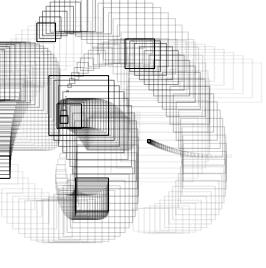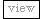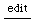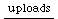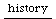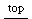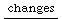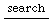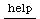Pen extensions

plotting 2D Arrays (shape [2,2])

class extension: extPlot2D.sc example code: array_plot2D.rtf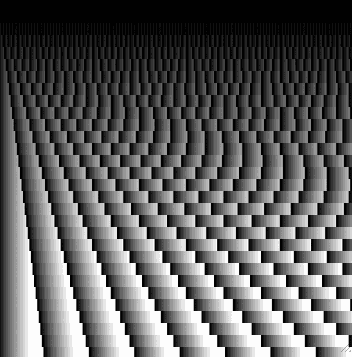Array.fill2D(30, 230, { arg i, j; j % i / 20 }).plot2D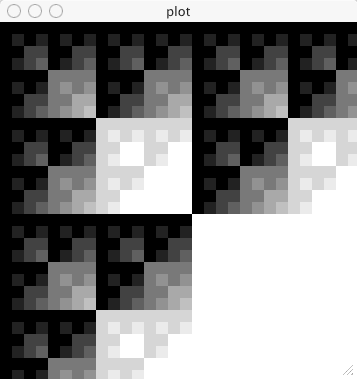Array.fill2D(30, 30, { arg i, j; (i & j)  / 10 }).plot2D

the "&" operator does a bitwise AND operation on the two numbers i and j. See boolean operations on signals or numbers

3D

class extension: extPen3D.sc example code: pen3Dtest.rtf pen3DtestAnimate.rtf